Reports 1-1 of 1
Injection system (General activities)
derossi, mantovani - 15:48 Tuesday 20 August 2019 (46720) Print this report
EIB scattered light analysis

Analyzing the signals during the injections made yesterday on the EIB (#46707) we found scattered light arches on PRCL in the frequency range 20-60 Hz, in particular for the longitudinal injections x and z (plots 1 to 4).

We ran the Bas's matlab script in order to exctrat the coupling factor. We found the typical scattered light structures (arches like) for the x and z movements of the EIB. The coupling factors are: Gx= 3.68695e-3^2 and Gz=3.56866e-3^2 (plots 5 and 6).

We then used Gx and Gz to make the projection on PRCL. We have refined the parameters on the ffts of the injections.

we computed the first 3 bounces by multiplying the EIB real movement Z = SBE_EIB_LVDT_x_200Hz (in micrometer) times 4*pi/1064e-9, taking the sine sin(4*pi/1064e-9*Z), and finally multiplying it by the coupling factor Gx.

The second bounce can be obtained by multiplying x 2 the path and reducing the coupling factor, the 3rd bounce multiplying x 3 the path and further reducing the coupling factor and so on...

For the x DOF, in order to overlap the 2nd and 3rd bounces on PRCL we had to multiply the path Z by a factor 1.1 (can be due to a not perfectly calibrated EIB signal) and set the coupling factors to Gx/1.2, Gx/2.4 and Gx/7.2 (plot 7).

For the z DOF in order to overlap the 2nd and 3rd bounces on PRCL we had to reduce the path by a factor 0.85 (thus 1.7 and 2.55 for the double and triple bounce). The coupling factors are respectively Gz, Gz/2 and Gz/6 (plot 8).

We then took a GPS quiet and tried to see if the structures visible on the PRCL error signal could be due to the scattering light from EIB (plots 9 and 10). The fact that they do not fit the PRCL curve could mean that the scattered light is modulated by the movement of the EIB during the injection, but its source is located somewhere else (maybe SIB1 or SIB2?).

We should plan other injections of the benches SIB1 and repeat the analysis to better understand where the scattered light comes from.

Images attached to this report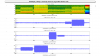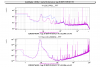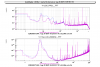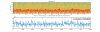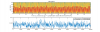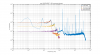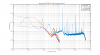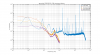×

×